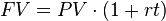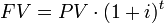## Defining the Time Value of Money

The Time Value of Money is the concept that money is worth more today that it is in the future.

### Learning Objectives

Identify the variables that are used to calculate the time value of money

### Key Takeaways

#### Key Points

• Being given $100 today is better than being given$100 in the future because you don’t have to wait for your money.
• Money today has a value ( present value, or PV) and money in the future has a value ( future value, or FV).
• The amount that the value of the money changes after one year is called the interest rate (i). For example, if money today is worth 10% more in one year, the interest rate is 10%.

#### Key Terms

• Present Value (PV): The value of the money today.
• Interest Rate (i or r): The cost of not having money for one period, or the amount paid on an investment per year.
• Future Value (FV): The value of the money in the future.

One of the most fundamental concepts in finance is the Time Value of Money. It states that money today is worth more than money in the future.

Imagine you are lucky enough to have someone come up to you and say “I want to give you $500. You can either have$500 right now, or I can give you $500 in a year. What would you prefer? ” Presumably, you would ask to have the$500 right now. If you took the money now, you could use it to buy a TV. If you chose to take the money in one year, you could still use it to buy the same TV, but there is a cost. The TV might not be for sale, inflation may mean that the TV now costs $600, or simply, you would have to wait a year to do so and should be paid for having to wait. Since there’s no cost to taking the money now, you might as well take it. There is some value, however, that you could be paid in one year that would be worth the same to you as$500 today. Say it’s $550- you are completely indifferent between taking$500 today and $550 next year because even if you had to wait a year to get your money, you think$50 is worth waiting.

In finance, there are special names for each of these numbers to help ensure that everyone is talking about the same thing. The $500 you get today is called the Present Value (PV). This is what the money is worth right now. The$550 is called the Future Value (FV). This is what $500 today is worth after the time period (t)- one year in this example. In this example money with a PV of$500 has a FV of $550. The rate that you must be paid per year in order to not have the money is called an Interest Rate (i or r). All four of the variables (PV, FV, r, and t) are tied together in the equation in. Don’t worry if this seems confusing; the concept will be explored in more depth later.Simple Interest Formula: Simple interest is when interest is only paid on the amount you originally invested (the principal). You don’t earn interest on interest you previously earned. ## Importance of the Time Value of Money Time value of money is integral in making the best use of a financial player’s limited funds. ### Learning Objectives Describe why the time value of money is important when analyzing a potential project ### Key Takeaways #### Key Points • Money today is worth more than the same quantity of money in the future. You can invest a dollar today and receive a return on your investment. • Loans, investments, and any other deal must be compared at a single point in time to determine if it’s a good deal or not. • The process of determining how much a future cash flow is worth today is called discounting. It is done for most major business transactions during investing decisions in capital budgeting. #### Key Terms • interest rate: The percentage of an amount of money charged for its use per some period of time. It can also be thought of as the cost of not having money for one period, or the amount paid on an investment per year. • discounting: The process of determining how much money paid/received in the future is worth today. You discount future values of cash back to the present using the discount rate. ### Why is the Time Value of Money Important? The time value of money is a concept integral to all parts of business. A business does not want to know just what an investment is worth today­it wants to know the total value of the investment. What is the investment worth in total? Let’s take a look at a couple of examples. Suppose you are one of the lucky people to win the lottery. You are given two options on how to receive the money. 1. Option 1: Take$5,000,000 right now.
2. Option 2: Get paid $600,000 every year for the next 10 years. In option 1, you get$5,000,000 and in option 2 you get $6,000,000. Option 2 may seem like the better bet because you get an extra$1,000,000, but the time value of money theory says that since some of the money is paid to you in the future, it is worth less. By figuring out how much option 2 is worth today (through a process called discounting), you’ll be able to make an apples-to-apples comparison between the two options. If option 2 turns out to be worth less than $5,000,000 today, you should choose option 1, or vice versa. Let’s look at another example. Suppose you go to the bank and deposit$100. Bank 1 says that if you promise not to withdraw the money for 5 years, they’ll pay you an interest rate of 5% a year. Before you sign up, consider that there is a cost to you for not having access to your money for 5 years. At the end of 5 years, Bank 1 will give you back $128. But you also know that you can go to Bank 2 and get a guaranteed 6% interest rate, so your money is actually worth 6% a year for every year you don’t have it. Converting our present cash worth into future value using the two different interest rates offered by Banks 1 and 2, we see that putting our money in Bank 1 gives us roughly$128 in 5 years, while Bank 2’s interest rate gives $134. Between these two options, Bank 2 is the better deal for maximizing future value.Compound Interest: In this formula, your deposit ($100) is PV, i is the interest rate (5% for Bank 1, 6% for Bank 2), t is time (5 years), and FV is the future value.